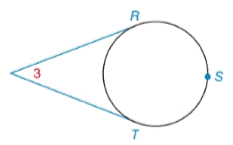Chapter 6.2, Problem 12EElementary Geometry For College St...

7th Edition
Alexander + 2 others
ISBN: 9781337614085

Solutions

Chapter
SectionElementary Geometry For College St...

7th Edition
Alexander + 2 others
ISBN: 9781337614085
Textbook Problem

Given: m ∠ 3 =42 ° Find: a) m R T ⌢ b) m R S T ⌢Exercises 12 ,   13

To determine

a)

To find:

mRT when m3=42°

Explanation

Theorem:

If an angle is formed by two intersecting tangents, then the measure of the angle is one-half the difference of the measures of its intercepted arcs.

Calculation:

m3=42°

mRT=ymRST=x

By Theorem

m3=12(mRSTmRT)42°=12(xy)x

To determine

b)

To find:

mRST when m3=42°

Still sussing out bartleby?

Check out a sample textbook solution.

See a sample solution

The Solution to Your Study Problems

Bartleby provides explanations to thousands of textbook problems written by our experts, many with advanced degrees!

Get Started